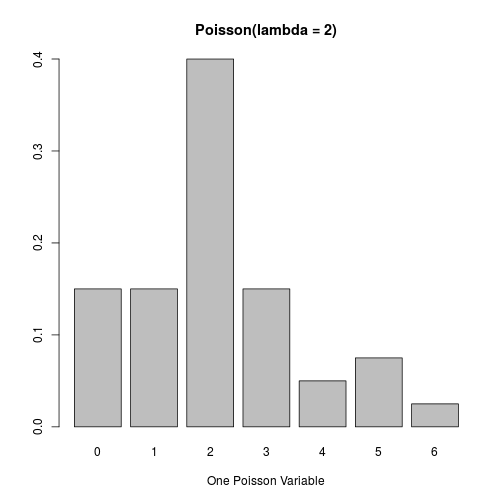## poisson-1.R
## Name: Paul E. Johnson <pauljohn at ku.edu>
## 2013-02-11
##
## Description: Draw a random sample from a Poisson distribution
## then plot it.  Debate value of histogram versus
## other plots

## Poisson is a "one parameter" distribution. That
## parameter is traditionally referred to by the Greek
## letter "lambda".  The expected value of the
## variable is equal to that parameter's value.
## The variance is also equal to lambda.

## I don't want to use a variable called "l" because that gets
## confused with the number "1". So I'm going to use "ell".

## you can adjust the ell and the sampleSize to see what
## happens

ell <- 2
sampleSize <- 40

z <- rpois(sampleSize, lambda = ell )

hist(z, prob= TRUE, main = paste("My Poisson(",ell,") Histogram."))## I think a histogram is the wrong type of plot for a discrete
## variable. It is wrong in lots of ways. Compare that with
## these two other ideas. We need
## a barplot or a special spike plot. This is better
t1 <- table(z)/sampleSize

plot(t1, type = "h", xlab = "A plot, type = \"h\"", main = paste("Poisson(lambda = ", ell,")", sep = ""))
## I'm straining to get the values for the points. I don't recall fighting
## with this in the past.
points(unique(as.numeric(names(t1))), as.vector(t1))##
barplot(t1, xlab = "One Poisson Variable", main = paste("Poisson(lambda = ", ell,")", sep = ""))## See what was so grossly wrong with the histogram?  Wrong Values!
## The first bar groups together outcomes 0 and 1! Booh!